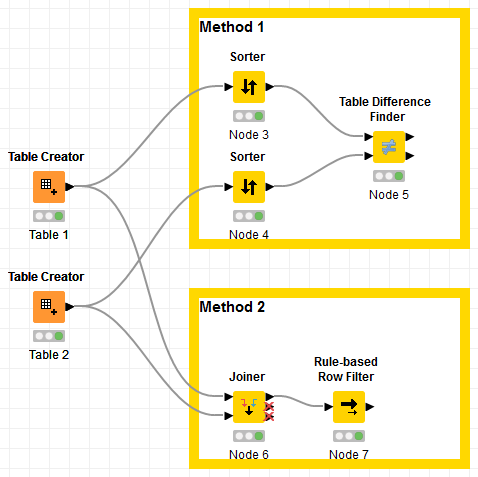# Compare two tables and get the row where values are different

I have two tables.

Table 1 (Col1 is primary key):

Col1 Col2 Col3
a a a
b b a
c b b

Table 2 (Col1 is primary key):

Col1 Col2 Col3
a b b
b b a
c a a

Resultant Table:

Col1 Col2 Col3
a a a
c a a

The row must be compared with respect to primary key and if there are different values in the respective column then filter out the rows.

How can I achieve this?

Hi @i_m_dps , and welcome to the Knime Community.

I get what you are trying to do, but I don’t understand the logic for the Resultant Table. We agree that the rows for “a” and “c” as primary key have different values in the 2 table, but from which table are the values for Col2 and Col3 are coming from for the Resultant Table? It looks like they came from Table 1 for “a”, and from Table 2 for “c” (row “a” has values a,a from Table 1, row “c” has values a,a from Table 2).

What is the logic for choosing values from Table 1 or Table 2 for the Resultant Table?

And do all primary keys from Table 1 exist in Table 2? And they’re primary keys in both tables (meaning no duplicates, and 1-to-1 relation)?

EDIT: Regardless of the logic (I’ll let you implement this part), I’ll give you 2 ways of doing this:

1. If the structures are the same on both tables and they have the same primary key, you can use the Table Difference Finder node:
Table Difference Finder – KNIME Hub
Just make sure that both tables are sorted the same way (the best way is to sort by the primary key on both)

2. You can use an inner join joining the 2 tables on Col1 (primary key) and getting the columns from both tables. Then use Rule-based Row Filter with the rule of Col2 from Table 1 not equal to Col2 from Table 2 and Col3 from Table 1 not equal to Col3 from Table 2

Here’s a quick workflow that contains both methods:Workflow: Get rows between 2 tables where values are different.knwf (17.5 KB)

3 Likes

Thanks a lot for the help.

I think I had made a mistake explaining the question. If the rows are different than it will take the row from first table. So, the resultant table will be

Col1 Col2 Col3
a a a
c b b

You can do a left join and then filter the rows which have a match
br

This topic was automatically closed 7 days after the last reply. New replies are no longer allowed.